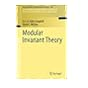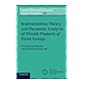Normal view MARC view ISBD view

# Modular invariant theory / H.E.A. Eddy Campbell, David L. Wehlau.

Material type:TextSeries: Encyclopaedia of mathematical sciences: v. 139.; Encyclopaedia of mathematical sciencesInvariant theory and algebraic transformation groups: 8.Publisher: Heidelberg ; New York : Springer, c2011Description: xiii, 233 p. : E 124.79 ill. ; 24 cm.ISBN: 9783642174032 (alk. paper); 3642174035 (alk. paper); 9783642174049 (eISBN).DDC classification: 512.23 Online resources: Inhaltsverzeichnis | Inhaltstext Summary: "This book covers the modular invariant theory of finite groups, the case when the characteristic of the field divides the order of the group. It explains a theory that is more complicated than the study of the classical non-modular case, and it describes many open questions. Largely self-contained, the book develops the theory from its origins up to modern results. It explores many examples, illustrating the theory and its contrast with the better understood non-modular setting. It details techniques for the computation of invariants for many modular representations of finite groups, especially the case of the cyclic group of prime order. It includes detailed examples of many topics as well as a quick survey of the elements of algebraic geometry and commutative algebra as they apply to invariant theory. The book is aimed at both graduate students and researchers--an introduction to many important topics in modern algebra within a concrete setting for the former, an exploration of a fascinating subfield of algebraic geometry for the latter"--back cover.
List(s) this item appears in: 2015-05-20
Item type Current location Call number Status Date due Barcode Item holds
Book Chennai Mathematical Institute
General Stacks
512.23 CAM (Browse shelf) Available 9528
Total holds: 0
##### Browsing Chennai Mathematical Institute Shelves , Shelving location: General Stacks Close shelf browser512.23 BLA Enumeration of finite groups / 512.23 BON Representations of SL2(Fq) / 512.23 BOR Mirrors and reflections : the geometry of finite reflection groups / 512.23 CAM Modular invariant theory / 512.23 CAR Cohomology rings of finite groups : with an appendix, calculations of cohomology rings of groups of order dividing 64 / 512.23 CEC Representation theory and harmonic analysis of wreath products of finite groups / 512.23 DAV The geometry and topology of coxeter groups /

Includes bibliographical references and index.

"This book covers the modular invariant theory of finite groups, the case when the characteristic of the field divides the order of the group. It explains a theory that is more complicated than the study of the classical non-modular case, and it describes many open questions. Largely self-contained, the book develops the theory from its origins up to modern results. It explores many examples, illustrating the theory and its contrast with the better understood non-modular setting. It details techniques for the computation of invariants for many modular representations of finite groups, especially the case of the cyclic group of prime order. It includes detailed examples of many topics as well as a quick survey of the elements of algebraic geometry and commutative algebra as they apply to invariant theory. The book is aimed at both graduate students and researchers--an introduction to many important topics in modern algebra within a concrete setting for the former, an exploration of a fascinating subfield of algebraic geometry for the latter"--back cover.